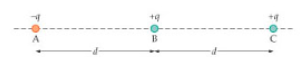# Problem: Consider the three electric charges, A, B, and C, shown in the figurePart A. Rank the charges in the order of decreasing magnitude of the net force they experience.Rank changes from largest to smallest magnitude of the net force they experience. To rank items as equivalent, overlap them.

###### FREE Expert Solution

In this problem, we are dealing with the electric force.

Electric force:

Coulomb's law:

$\overline{){\mathbf{F}}{\mathbf{=}}\frac{\mathbf{k}{\mathbf{q}}_{\mathbf{1}}{\mathbf{q}}_{\mathbf{2}}}{{\mathbf{r}}^{\mathbf{2}}}}$

83% (64 ratings)###### Problem Details

Consider the three electric charges, A, B, and C, shown in the figurePart A. Rank the charges in the order of decreasing magnitude of the net force they experience.Rank changes from largest to smallest magnitude of the net force they experience. To rank items as equivalent, overlap them.